﻿ 基于Swish激活函数的人脸情绪识别的深度学习模型研究 A Research on Deep Learning Model for Face Emotion Recognition Based on Swish Activation Function

Journal of Image and Signal Processing
Vol. 08  No. 03 ( 2019 ), Article ID: 31134 , 11 pages
10.12677/JISP.2019.83016

A Research on Deep Learning Model for Face Emotion Recognition Based on Swish Activation Function

Lingjiao Wang, Qian Li, Hua Guo

College of Information Engineering, Xiangtan University, Xiangtan Hunan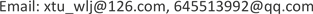Received: Jun. 11st, 2019; accepted: Jun. 27th, 2019; published: Jul. 3rd, 2019ABSTRACT

In recent years, deep learning model has been developed rapidly. As one of the methods, deep convolution neural network has been widely used in computer vision. There are many factors affecting the performance of deep learning model, among which the selection of activation function and the structure of neural network have important impact on the performance of deep learning model. This paper analyses the advantages and disadvantages of the traditional activation function and the new Swish activation function, introduces Swish function into the deep learning model of facial emotion, proposes an improved back propagation algorithm, and uses multi-layer small-size convolution module instead of large-size convolution module in the convolution neural network to extract refinement features, and constructs a new deep learning model of facial emotion recognition, Swish-FER-CNNs. The experimental results show that the recognition accuracy of deep learning model based on Swish activation function is higher than that of activation functions such as ReLU, L-ReLU and P-ReLU. With the improved network structure, the recognition accuracy of the deep learning model of Swish-FER-CNNS constructed in the paper is improved by 4.02% compared with the existing model.

Keywords:Activation Function, Back Propagation, Convolutional Neural Network, Deep Learning, Computer Vision1. 引言

2. 基于卷积神经网络的情绪识别模型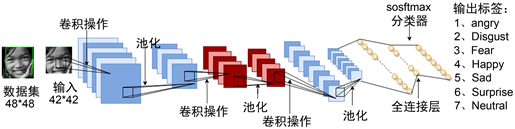Figure 1. Principle diagram of emotion recognition model

2.1. 反向传播算法

$\frac{\text{d}z}{\text{d}x}=\frac{\text{d}z}{\text{d}y}\frac{\text{d}y}{\text{d}x}$ (1)

2.1.1. 激活函数分析

$f\left(x\right)=\frac{1}{1+{\text{e}}^{-x}}$ (2)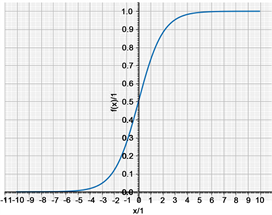Figure 2. Sigmoid activation function

$f\left(x\right)=\mathrm{max}\left(0,x\right)$ (3)

ReLU激活函数在 $x>0$ 的定义域内导数恒为定值，反向传播时可简化计算，加快收敛速度。在 $x<0$ 定义域内具有硬饱和特性：输入落在此区域，对应的输出皆为0，神经元反向传播一阶梯度亦为0，神经元不具有激活作用，即神经元死亡，导致模型的拟合力下降。此外，ReLU函数在 $x<0$ 定义域对应输出为0这一特性导致神经元输出均值大于0，不利于迭代计算，此问题被称为均值偏移：后一个神经元的输入为前一个神经元的输出，因输出皆为正值，后一个神经元的输入被限制，模型的拟合能力下降，制约深度模型的性能。

Maas等人引入L-ReLU激活函数可有效解决均值偏移问题，其函数定义为：

$f\left(x\right)=\left\{\begin{array}{l}x,\text{\hspace{0.17em}}\text{\hspace{0.17em}}\text{\hspace{0.17em}}\text{\hspace{0.17em}}x\ge 0\\ \alpha x,\text{\hspace{0.17em}}\text{\hspace{0.17em}}x<0\end{array}$ (4)

L-ReLU激活函数在 $x\ge 0$ 定义域，一阶导数恒定，利于计算，与ReLU性质一致。在 $x<0$ 定义域内，L-ReLU图像位于y轴的负半轴，减缓了均值偏移。

He Kaiming等人引入P-ReLU激活函数，以获取更贴合模型的负轴斜率，其函数定义为：

$f\left(x\right)=\left\{\begin{array}{l}x,\text{\hspace{0.17em}}\text{\hspace{0.17em}}\text{\hspace{0.17em}}\text{\hspace{0.17em}}\text{\hspace{0.17em}}x\ge 0\\ {\alpha }_{i}x,\text{\hspace{0.17em}}\text{\hspace{0.17em}}\text{ }x<0\end{array}$ (5)

$f\left(x\right)=\alpha *x*sigmoid\left(x\right)$ (6)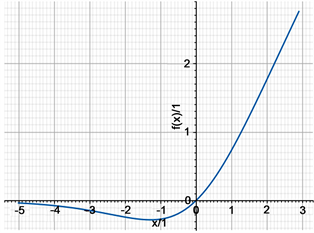Figure 3. Swish activation function

$x>0$ 时，Swish激活函数的一阶导数易于计算，利于模型训练。当 $x<0$ 时，与ReLU函数相比，Swish函数既能够均衡正负轴比重，减缓了均值偏移现象，又由于它无硬饱和性，避免了神经元死亡现象；与L-ReLU函数相比，Swish函数是非线性的，具有软饱和性，鲁棒性更好；与P-ReLU相比，Swish函数不需要计算参数 $\alpha$ ，减少了计算量，且鲁棒性更好。因此，Swish激活函数的性能优于ReLU、L-ReLU和P-ReLU函数。

2.1.2. Swish-FER-CNNs中的反向传播算法

${u}^{\left(i\right)}=\text{Swish}\left({Α}^{\left(i\right)}\right)$ (7)

$\frac{\partial {u}^{\left(n\right)}}{\partial {u}^{\left(i\right)}}=\sum _{i,j\in Pa\left({u}^{\left(i\right)}\right)}\frac{\partial {u}^{\left(n\right)}}{\partial {u}^{\left(i\right)}}\frac{\partial {u}^{\left(i\right)}}{\partial {u}^{\left(j\right)}}$ (8)

2.2. Swish-FER-CNNs网络模型

Table 1. Swish-FER-CNNs network architecture

3. 数据集

4. 实验分析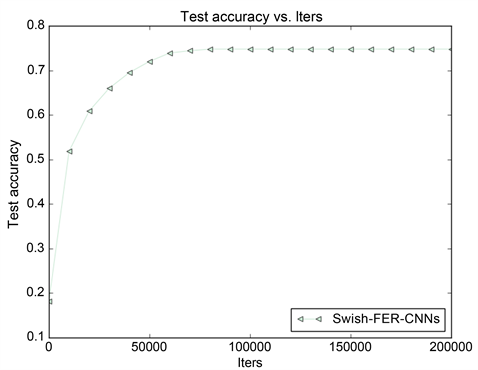Figure 4. Training accuracy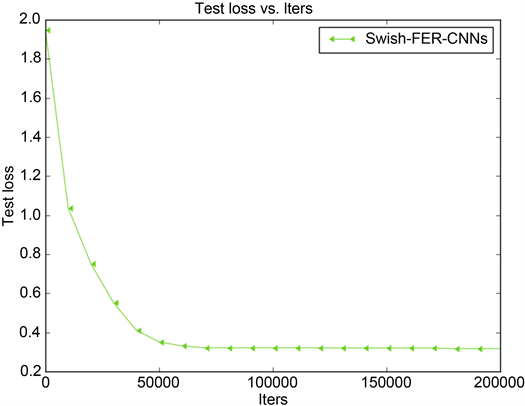Figure 5. Training loss function

${P}_{i}=\frac{T{P}_{i}}{T{P}_{i}+F{N}_{i}}=\frac{T{P}_{i}}{Su{m}_{i}}$ (9)

Swish-FER-CNNs学习模型对各类情绪识别的准确率如表2所示。Table 2. Confusion matrix of emotion recognition accuracy of Swish-FER-CNNs model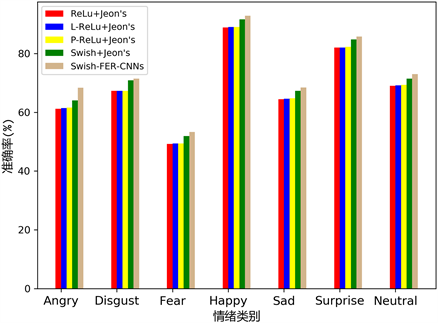Figure 6. Accuracy of test confusion matrix for each model(10)

5. 结束语

A Research on Deep Learning Model for Face Emotion Recognition Based on Swish Activation Function[J]. 图像与信号处理, 2019, 08(03): 110-120. https://doi.org/10.12677/JISP.2019.83016

1. 1. Giannopoulos, P., Perikos, I. and Hatzilygeroudis, I. (2018) Deep Learning Approaches for Facial Emotion Recognition: A Case Study on FER-2013. In: Hatzilygeroudis, I. and Palade, V., Eds., Advances in Hybridization of Intelligent Methods, Smart Innovation, Systems and Technologies, Springer, Cham, 1-16.
https://doi.org/10.1007/978-3-319-66790-4_1

2. 2. Bruce, V. and Young, A. (1986) Understanding Face Recognition. British Journal of Psychology, 77, 305-327.
https://doi.org/10.1111/j.2044-8295.1986.tb02199.x

3. 3. Ioffe, S. and Szegedy, C. (2015) Batch Normalization: Accelerating Deep Network Training by Reducing Internal Covariate Shift. Arxiv: 1502.03167.

4. 4. Simonyan, K. and Zisserman, A. (2014) Very Deep Convolutional Networks for Large-Scale Image Recognition. Arxiv: 1409.1556.

5. 5. Krizhevsky, A., Sutskever, I. and Hinton, G.E. (2012) ImageNet Classification with Deep Convolutional Neural Networks. In: Pereira, F., Burges, C.J.C., Bottou, L. and Weinberger, K.Q., Eds., Advances in Neural Information Processing Systems, The MIT Press, ‎Cambridge, MA, 1097-1105.

6. 6. Zhang, C. and Woodland, P.C. (2016) DNN Speaker Adaptation Using Parameterised Sigmoid and ReLU Hidden Activation Functions. 2016 IEEE International Conference on Acoustics, Speech and Signal Processing, Shanghai, 20-25 March 2016, 5300-5304.
https://doi.org/10.1109/ICASSP.2016.7472689

7. 7. Taigman, Y., Yang, M., Ranzato, M.A. and Wolf, L. (2014) Deepface: Closing the Gap to Human-Level Performance in Face Verification. 2014 IEEE Conference on Computer Vision and Pattern Recognition, Columbus, OH, 23-28 June 2014, 1701-1708.
https://doi.org/10.1109/CVPR.2014.220

8. 8. Szegedy, C., Ioffe, S., Vanhoucke, V. and Alemi, A.A. (2017) Inception-v4, Inception-Resnet and the Impact of Residual Connections on Learning. Proceedings of the Thirty-First AAAI Conference on Artificial Intelligence, San Francisco, CA, 4-9 February 2017, 4278-4284.

9. 9. Wang, X., Girshick, R., Gupta, A. and He, K. (2018) Non-Local Neural Networks. 2018 IEEE Conference on Computer Vision and Pattern Recognition, Salt Lake City, UT, 18-23 June 2018, 7794-7803.
https://doi.org/10.1109/CVPR.2018.00813

10. 10. Jeon, J., Park, J.C., Jo, Y.J., et al. (2016) A Real-Time Facial Expression Rec-ognizer Using Deep Neural Network. In: Proceedings of the 10th International Conference on Ubiquitous Information Management and Communication, ACM, New York, 94:1-94:4.
https://doi.org/10.1145/2857546.2857642

11. 11. LeCun, Y., Boser, B.E., Denker, J.S., et al. (1990) Handwritten Digit Recognition with a Back-Propagation Network. In: Pereira, F., Burges, C.J.C., Bottou, L. and Weinberger, K.Q., Eds., Advances in Neural Information Processing Systems, The MIT Press, ‎Cambridge, MA, 396-404.

12. 12. Ramachandran, P., Zoph, B. and Le, Q.V. (2017) Searching for Activation Functions. Computer Science, ArXiv: 1710.05941.

13. 13. Saxe, A.M., McClelland, J.L. and Ganguli, S. (2013) Exact Solutions to the Nonlinear Dynamics of Learning in Deep Linear Neural Networks. Computer Science, ArXiv: 1312.6120.

14. 14. Eger, S., Youssef, P. and Gurevych, I. (2019) Is It Time to Swish? Comparing Deep Learning Activation Functions Across NLP Tasks. Computer Science, arXiv: 1901.02671.

15. 15. Maas, A.L., Hannun, A.Y. and Ng, A.Y. (2013) Rectifier Nonlinearities Improve Neural Network Acoustic Models. International Conference on Machine Learning.

16. 16. Xu, B., Wang, N., Chen, T. and Li, M. (2015) Empirical Evaluation of Rectified Activations in Convolutional Network. Computer Science, arXiv: 1505.00853.

17. 17. Zhang, X., Zou, Y. and Shi, W. (2017) Dilated Convolution Neural Network with LeakyReLU for Environmental Sound Classification. 2017 22nd International Conference on Digital Signal Processing, London, 23-25 August 2017, 1-5.
https://doi.org/10.1109/ICDSP.2017.8096153

18. 18. He, K., Zhang, X., Ren, S. and Sun, J. (2015) Delving Deep into Rectifiers: Surpassing Human-Level Performance on ImageNet Classification.2015 IEEE International Conference on Computer Vision, Santiago, Chile, 7-13 December 2015, 1026-1034.
https://doi.org/10.1109/ICCV.2015.123

19. 19. Gulcehre, C., Moczulski, M., Denil, M. and Bengio, Y. (2016) Noisy Activation Functions. In: Proceedings of the 33rd International Conference on Machine Learning, PMLR 48, 3059-3068.

20. 20. Jia, Y., Shelhamer, E., Donahue, J., et al. (2014) Caffe: Convolutional Architecture for Fast Feature Embedding. In: Balcan, M.F. and Weinberger, K.Q., Eds., Proceedings of the 22nd ACM International Conference on Multimedia, ICML, New York, 675-678.